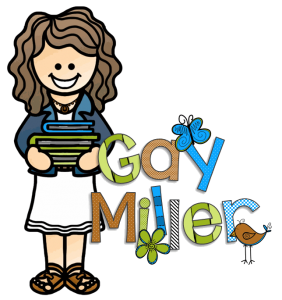# Coordinate Grid ActivitiesThis coordinate grid foldable organizer with building pieces can be used for many activities:

• First students plan a town and then write the coordinates for each location.

• Students can determine how many blocks from one location to another.

• Students can write directions from one location to another using coordinate pairs to indicate turns.

• Have students work in small groups. One student plans a town and then calls out the coordinates for other students to “build” the same town. When finished students can compare to see if all the “towns” match.

• Follow instructions to find a “mystery” location.

This activity is part of The Number System found at Teachers Pay Teachers.

## Links to Online Interactive Resources

• Graphing Worksheets [This website has four quadrant graphing puzzles with cute characters for different holidays.]

## Coordinate Grid Activity

Students used the layout of the floor tiles of move to the correct locations of the life-sized coordinate grid. FUN!## Common Core Standards

CCSS.Math.Content.5.G.A.1
Use a pair of perpendicular number lines, called axes, to define a coordinate system, with the intersection of the lines (the origin) arranged to coincide with the 0 on each line and a given point in the plane located by using an ordered pair of numbers, called its coordinates. Understand that the first number indicates how far to travel from the origin in the direction of one axis, and the second number indicates how far to travel in the direction of the second axis, with the convention that the names of the two axes and the coordinates correspond (e.g., x-axis and x-coordinate, y-axis and y-coordinate).Classify two-dimensional figures based on the presence or absence of parallel or perpendicular lines, or the presence or absence of angles of a specified size. Recognize right triangles as a category, and identify right triangles.

CCSS.Math.Content.5.G.A.2
Represent real world and mathematical problems by graphing points in the first quadrant of the coordinate plane, and interpret coordinate values of points in the context of the situation.

CCSS.Math.Content.6.G.A.3
Draw polygons in the coordinate plane given coordinates for the vertices; use coordinates to find the length of a side joining points with the same first coordinate or the same second coordinate. Apply these techniques in the context of solving real-world and mathematical problems.